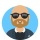Home Communities
IT Knowledge
Inspiration
Languages
EN

# JavaScript - how can I convert array of strings to array of objects?

6 pointsAaron1
448

I have this specific problem.

``````// I have:
var array = ['x', 'y'];

// I need to convert above array to below object.
// Frist object needs to have 2 properties 'a' and 'b' with same value from array
// Second object needs to have 2 properties 'a' and 'b' with same value from array
// result:
var objects = [
{
'a': 'x',
'b': 'x'
},
{
'a': 'y',
'b': 'y'
}
];``````

I know how to convert it with simple for loop.

How to do this in the better way?

5 pointsAaron1
448

Quick solution using map function:

``````// ONLINE-RUNNER:browser;

var array = ['x', 'y'];

var objects = array.map(value => (
{
'a': value,
'b': value
}
));

console.log(JSON.stringify(objects)); // [{"a":"x","b":"x"},{"a":"y","b":"y"}]``````

Solution using forEach:

``````// ONLINE-RUNNER:browser;

var array = ['x', 'y'];
var objects = [];

array.forEach(value => objects.push({ 'a': value, 'b': value }));

console.log(JSON.stringify(objects)); // [{"a":"x","b":"x"},{"a":"y","b":"y"}]``````

Implementation you mentioned in your question, using simple for:

``````// ONLINE-RUNNER:browser;

var array = ['x', 'y'];
var objects = [];

for (var i = 0; i < array.length; i++) {
objects.push({ 'a': array[i], 'b': array[i] });
}

console.log(JSON.stringify(objects)); // [{"a":"x","b":"x"},{"a":"y","b":"y"}]``````